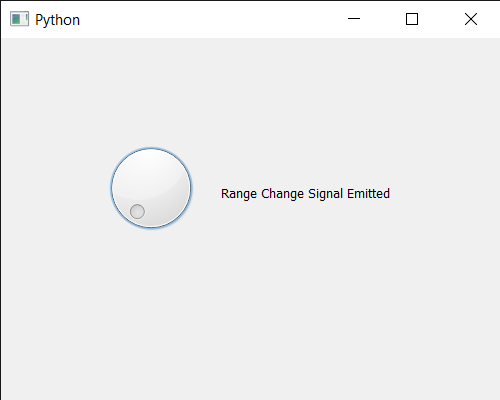# PyQt5 QDial – Range Changed Signal

• Last Updated : 07 Jul, 2020

In this article we will see how we get the range change signal of the QDial. This signal is emitted when the slider range has changed, by default the range of QDial is 0 to 99 although we can change this any time with the help of `setRange` method.

In order to do this we use `rangeChanged` method with the QDial object

Attention geek! Strengthen your foundations with the Python Programming Foundation Course and learn the basics.

To begin with, your interview preparations Enhance your Data Structures concepts with the Python DS Course. And to begin with your Machine Learning Journey, join the Machine Learning - Basic Level Course

Syntax : dial.rangeChanged.connect(method)

Argument : It takes method as argument

Return : It returns None

Below is the implementation

 `# importing libraries``from` `PyQt5.QtWidgets ``import` `*` `from` `PyQt5 ``import` `QtCore, QtGui``from` `PyQt5.QtGui ``import` `*` `from` `PyQt5.QtCore ``import` `*` `import` `sys`` ` ` ` `class` `Window(QMainWindow):`` ` `    ``def` `__init__(``self``):``        ``super``().__init__()`` ` `        ``# setting title``        ``self``.setWindowTitle(``"Python "``)`` ` `        ``# setting geometry``        ``self``.setGeometry(``100``, ``100``, ``500``, ``400``)`` ` `        ``# calling method``        ``self``.UiComponents()`` ` `        ``# showing all the widgets``        ``self``.show()`` ` `    ``# method for components``    ``def` `UiComponents(``self``):`` ` `        ``# creating QDial object``        ``dial ``=` `QDial(``self``)`` ` `        ``# setting geometry to the dial``        ``dial.setGeometry(``100``, ``100``, ``100``, ``100``)`` ` `        ``# creating a label``        ``label ``=` `QLabel(``"GeeksforGeeks"``, ``self``)`` ` `        ``# setting geometry to the label``        ``label.setGeometry(``220``, ``125``, ``200``, ``60``)`` ` `        ``# making label multiline``        ``label.setWordWrap(``True``)`` ` `        ``# range changed signal``        ``dial.rangeChanged.connect(``lambda``: label.setText(``"Range Change Signal Emitted"``))`` ` `        ``# setting range of dial``        ``dial.setRange(``0``, ``1000``)`` ` `# create pyqt5 app``App ``=` `QApplication(sys.argv)`` ` `# create the instance of our Window``window ``=` `Window()`` ` `# start the app``sys.exit(App.``exec``())`

Output :My Personal Notes arrow_drop_up